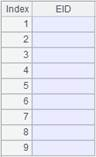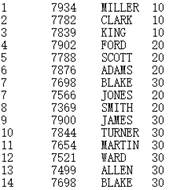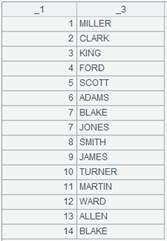# groupn()

## cs.groupn(x;C)

cs.groupn(x;C)

cs游标记录根据x分组后，推入管道序列C中的管道。

 cs 游标记录 x 分组表达式 C 管道序列

 A 1 =demo.cursor("select NAME,GENDER,DEPT,BIRTHDAY from EMPLOYEE") 2 =channel() 创建管道 3 =channel() 创建管道 4 =A1.groupn(if(GENDER=="F",1,2);[A2,A3]) 将游标记录分组，GENDER值为F为一组推送到管道A2中，其余的记录分为另一组推送到管道A3中 5 =A2.fetch() 保留管道当前数据 6 =A3.fetch() 保留管道当前数据 7 =A1.fetch() 8 =A2.result() 获取管道A2计算结果9 =A3.result() 获取管道A3计算结果## ch.groupn(x;C)

ch.groupn(x;C)

ch管道中的记录根据x分组后，推入管道序列C中的管道。

 ch 管道 x 分组表达式 C 管道序列

 A 1 =demo.cursor("select NAME,GENDER,DEPT,BIRTHDAY from EMPLOYEE") 2 =channel(A1) 将游标记录推入管道，结果返回管道 3 =channel() 创建管道 4 =channel() 创建管道 5 =A2.groupn(if(GENDER=="F",1,2);[A3,A4]) 将管道A2中的记录分组，GENDER值为F为一组推送到管道A3中，其余的记录分为另一组推送到管道A4中 6 =A3.fetch() A3管道附加结果集函数ch.fetch()，保留管道A3的当前数据 7 =A4.fetch() A4管道附加结果集函数ch.fetch()，保留管道A4的当前数据 8 =A1.fetch() 9 =A3.result() 获取管道A3计算结果10 =A4.result() 获取管道A4计算结果## cs.groupn(x;F)

cs.groupn(x;F)

 cs 游标记录 x 分组表达式 F 集文件对象序列

 A 1 =demo.cursor("select NAME,GENDER,DEPT,BIRTHDAY from EMPLOYEE") 2 =[file("f_dept.btx"),file("m_dept.btx")] 集文件对象序列 3 =A1.groupn(if(GENDER=="F",1,2);A2) 将GENDER值为F的记录分到第1组，其余的记录分到第2组，并将分组子集写入到对应顺序的集文件中 4 =A3.fetch()